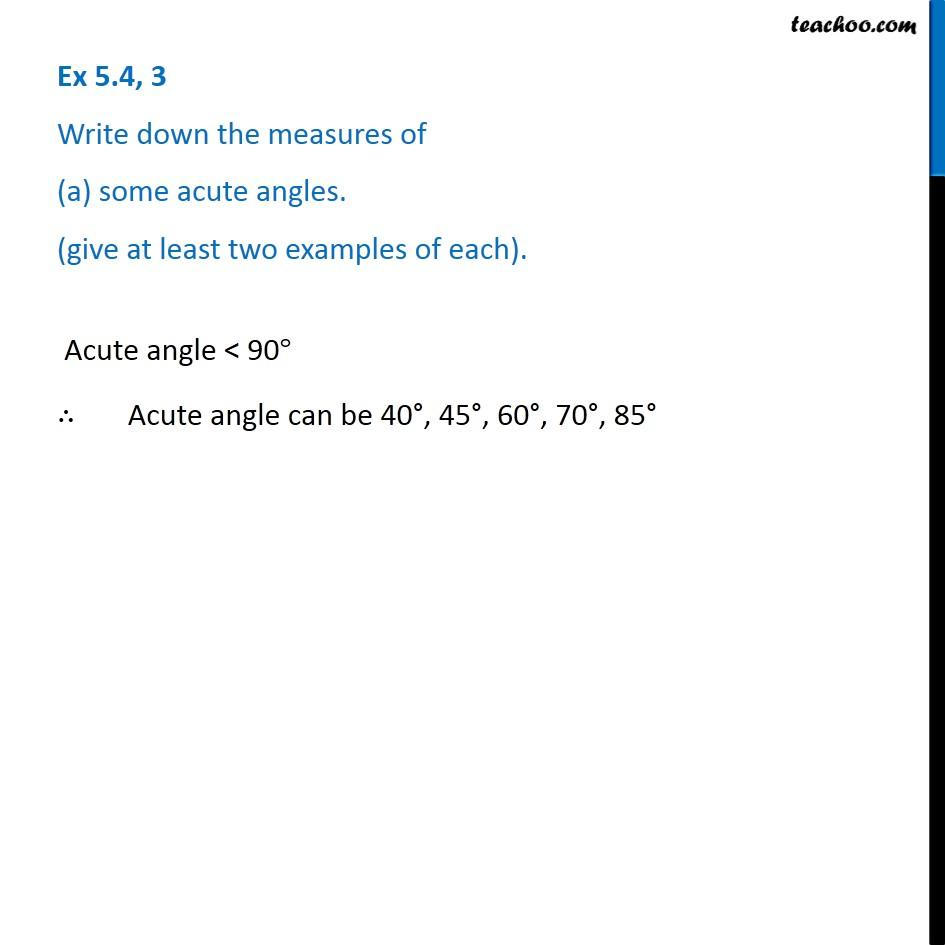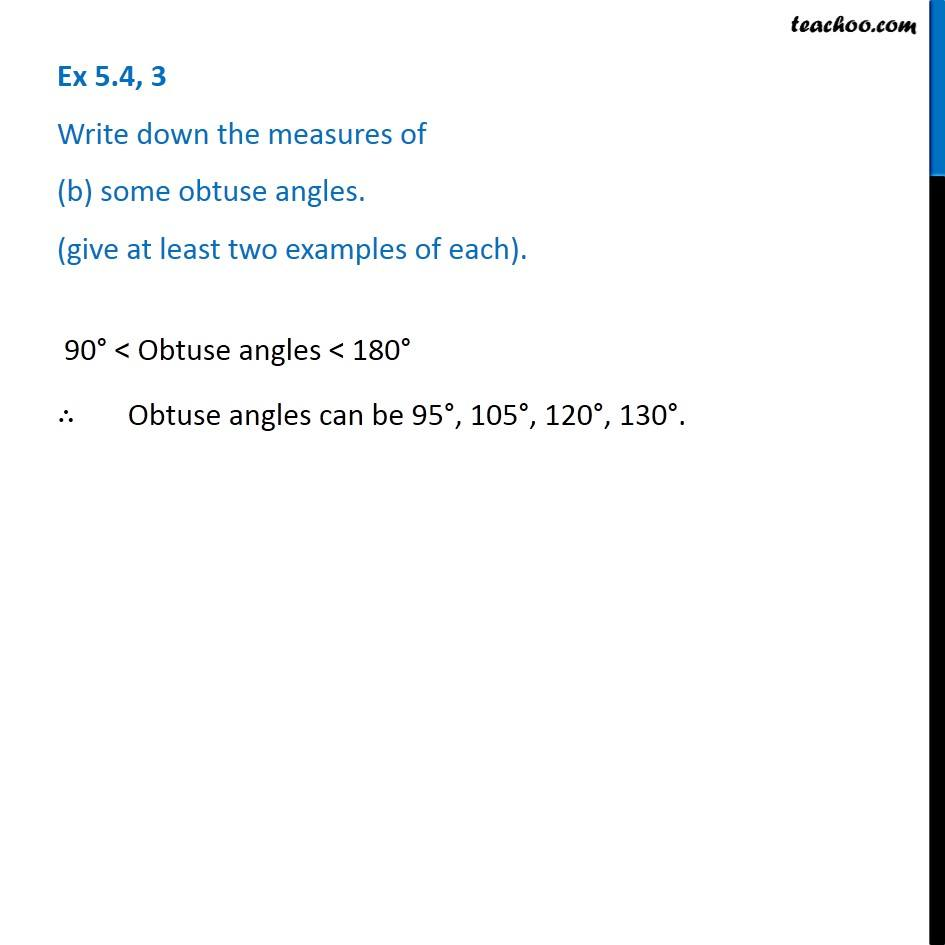Ex 5.4

Chapter 5 Class 6 Understanding Elementary Shapes
Serial order wise### Transcript

Ex 5.4, 3 Write down the measures of (a) some acute angles. (give at least two examples of each). Acute angle < 90° ∴ Acute angle can be 40°, 45°, 60°, 70°, 85° Ex 5.4, 3 Write down the measures of (b) some obtuse angles. (give at least two examples of each). 90° < Obtuse angles < 180° ∴ Obtuse angles can be 95°, 105°, 120°, 130°.